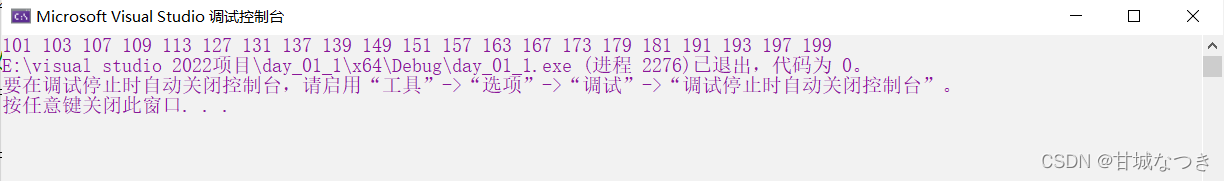# 【C语言系列】— 打印100~200之间的素数

​​​​​​​

꧁   各位大佬们好！很荣幸能够得到您的访问，让我们一起在编程道路上任重道远！꧂

☙ 博客专栏：【C生万物】

⛅ 本篇内容简介：C语言实现100~200之间素数的打印！

⭐ 了解作者：励志成为一名编程大牛的学子，目前正在升大二的编程小白。

✂   正文开始

## 解题思路

3.直接上手个代码看吧：

``````#define _CRT_SECURE_NO_WARNINGS 1
//打印100~200之间的素数
#include<stdio.h>
int main()
{
int i = 0;//先定义一个i，为后的循环做准备
for (i = 100; i <= 200; i++)//定义一个for循环
{
int j = 0;//定义一个用于与100-200之间的数相除
for (j = 2; j <= i; j++)//用大于2，小于i的j取模
{
if (i % j == 0)//若i能被j整除就跳出循环
{
break;
}
}
if (j>=i)//j一直找，直到找到大于i的数都没有找到整除的数，就是素数
{
printf("%d ", i);//把素数打印出来
}
}
return 0;
}``````4.大家都知道偶数不可能是质数所以我们可以进一步的取修改我们的代码：本来是i++的，可以修改为i+2 :

``````#include<stdio.h>
int main()
{
int i = 0;//先定义一个i，为后的循环做准备
for (i = 101; i <= 200; i+=2)//定义一个for循环
{
int j = 0;//定义一个用于与100-200之间的数相除
for (j = 2; j <= i; j++)//用大于2，小于i的j取模
{
if (i % j == 0)//若i能被j整除就跳出循环
{
break;
}
}
if (j>=i)//j一直找，直到找到大于i的数都没有找到整除的数，就是素数
{
printf("%d ", i);//把素数打印出来
}
}
return 0;
}``````

（还只是写博客的新手，不对的大家可以帮忙指出，修改）